# How to easily remember trigonometric ratios table

Trigonometric ratios table for standard angles is given as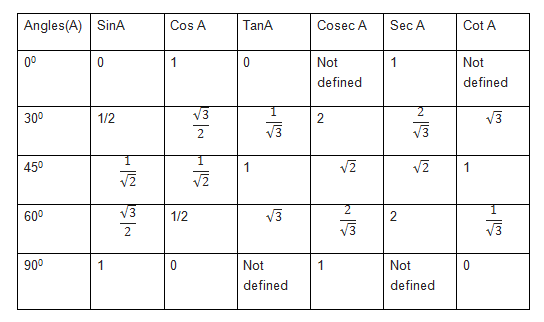Students often find it difficult to remember and use it trigonometric equations, questions and problems

There is one easy way to remember these trigonometric ratios table.Lets check it out

1.For sin ratios for 0,30 ,45 ,60 and 90, we can remember them as

$sin 0 = \sqrt { \frac {0}{4}} = 0$

$sin 30 = \sqrt {\frac {1}{4}} = 1/2$

$sin 45 = \sqrt {\frac {2}{4}} = \frac {1}{ \sqrt {2}}$

$sin 60 = \sqrt { \frac {3}{4}} = \frac {\sqrt {3}}{2}$

$sin 90 = \sqrt {\frac {4}{4}} =1$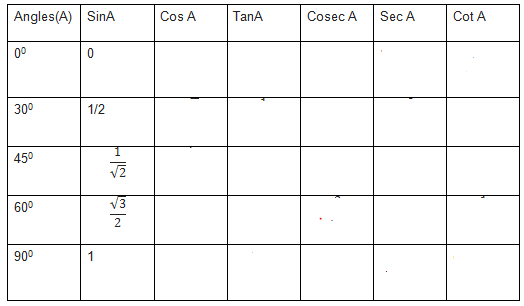2.for cos ratios for 0,30 ,45 ,60 and 90, we can remember them as opposite order from sin 90 to 0

$cos 0 = sin 90 =1$

$cos 30 =sin 60 = \frac {\sqrt {3}}{2}$

$cos 45= sin45 =\frac {1}{ \sqrt {2}}$

$cos 60 =sin 30 = 1/2$

$cos 90 =sin 0 =0$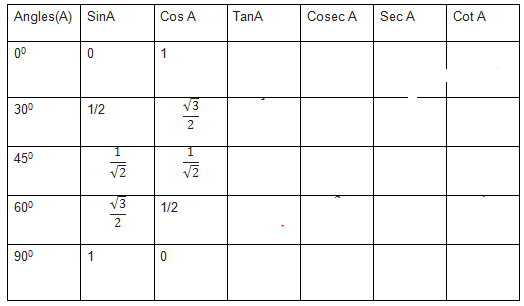3.for tan ratios for 0,30 ,45 ,60 and 90, we can remember them as

$tan x = \frac {sin x}{cos x}$

$tan 0 = 0/1 =0$

$tan 30 = \frac {sin 30}{cos 30}= \frac {1}{ \sqrt {3}}$

$tan 45 = \frac {sin 45}{cos 45}= 1$

$tan 60 = \frac {sin 60}{cos 60}= \sqrt {3}$

$tan 90 = \frac {sin 90}{cos 90}= 1/0$ .This is undefined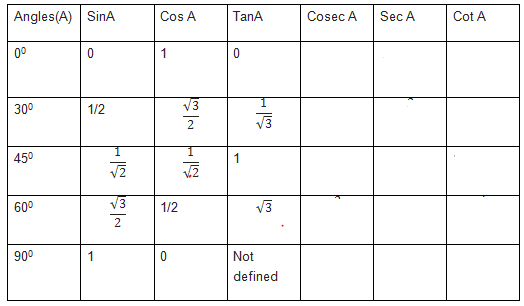1. For cosec ratios for 0,30 ,45 ,60 and 90, we can remember them as

$cosec A = \frac {1}{sinA}$

$cosec 0 = 1/0$ .This is undefined

$cosec 30 = \frac {1}{sin30} =2$

$cosec 45 = \frac {1}{sin45} =\sqrt {2}$

$cosec 60 = \frac {1}{sin60} =\frac {2} {\sqrt {3}}$

$cosec 90 = \frac {1}{sin90} =1$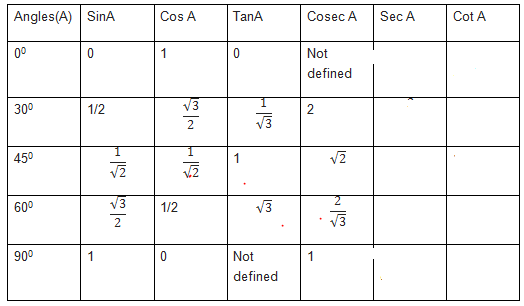5.for sec ratios for 0,30 ,45 ,60 and 90, we can remember them as

$sec A = \frac {1}{cosA}$

$sec 0 = 1$ .

$sec 30 = \frac {1}{cos30} =\frac {2} {\sqrt {3}}$

$cosec 45 = \frac {1}{cos45} =\sqrt {2}$

$cosec 30 = \frac {1}{sin60} =2$

$cosec 90 = \frac {1}{cos90} =1/0$. This is undefined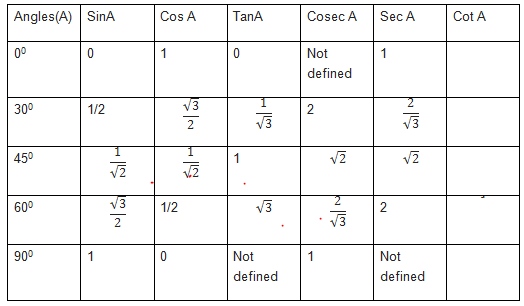6.for cot ratios for 0,30 ,45 ,60 and 90, we can remember them as

$cot x = \frac {cos x}{sin x}$

$cot 0 = 1/0$ This is undefined

$cot 30 = \sqrt {3}$

$cot 45 = 1$

$tan 60 = \frac {1}{ \sqrt {3}}$

$cot 90 = \frac {cos 90}{sin 90}= 0$ .

Related Articles

Trigonometric table from 0 to 360 (cos -sin-cot-tan-sec-cosec)

Trigonometry Formulas for class 11 (PDF download)

trigonometric formulas for Class 10

Important trigonometry questions for class 11 Maths